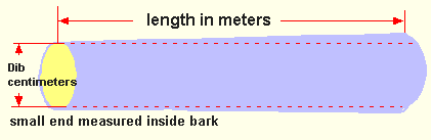Spike's Calculators

# Cubic Metre Rule CalculatorCalculate the volume of a log in cubic metres for diameter in centimetres and length in metres.Calculate the volume of a log in cubic metres for diameter in centimetres and length in metres.

Diameters are measured inside the bark in 2 centimetre size class intervals, with the class boundary occurring on the odd centimetre, and recorded in even centimetre classes.

A scaled log that coincides with the class boundary of two size classes belongs to the lower size class.

Example: A diameter that falls on the class boundary between 10 and 12 centimetres (cm) must be read as 10 centimetres.

Diameter Measured Inside Bark = DIB